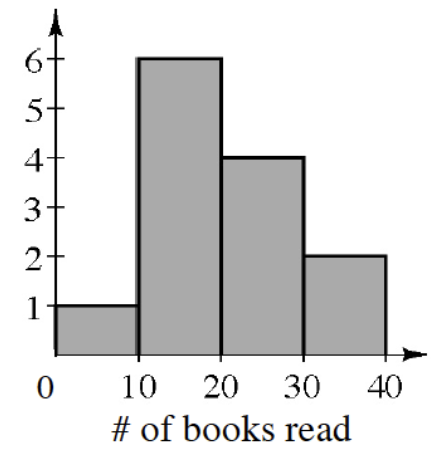### Home > CC1 > Chapter 8 > Lesson 8.1.5 > Problem8-67

8-67.

Create a histogram of the data from problem 8-66. What are the advantages and disadvantages of displaying the data using a histogram?

What does a histogram allow you to visually determine that other plots do not?

Compare the histogram with the stem-and-leaf plot from problem 8-66.$\begin{array}{l|l} \text{Note: 4} & \ 1 \text{ means }41 \quad \end{array}$

$\begin{array}{r|l} 0 & 9 \\ 1 & 0, 2, 4, 7, 7, 8\\ 2 & 0, 1, 4, 5\\ 3 & 0, 1 \end{array}$